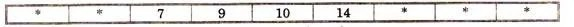Median: Characteristics, Applications and Limitations

8th February 2020

The median of a set of data values is the middle value of the data set when it has been arranged in ascending order. That is, from the smallest value to the highest value.

Example:

The marks of nine students in a geography test that had a maximum possible mark of 50 are given below:

47     35     37     32     38     39     36     34     35

Find the median of this set of data values.

Solution:

Arrange the data values in order from the lowest value to the highest value:

32     34     35     35     36     37     38     39     47

The fifth data value, 36, is the middle value in this arrangement.

Merits or Uses of Median:

1. Median is rigidly defined as in the case of Mean.
2. Even if the value of extreme item is much different from other values, it is not much affected by these values e.g. Median in case of 4, 7, 12, 18, 19 is 12 and if we add two values equal to 450 10000, new median is 18.
3. It can also be used for the Quantities; those can’t give A.M; as is in case of intelligence etc. It is possible to arrange in any order and to locate the middle valve. For such cases it is the best measure.
4. It can be located graphically.
5. For open end intervals, it is also suitable one. As taking any value of the intervals, value of Median remains the same.
6. It can be easily calculated and is also easy to understand
7. Median is also used for other statistical devices such as Mean Deviation and skewness.
8. It can be located by inspection in some cases.
9. Extreme items may not be available to get Median. Only if number of terms is known, we can get median e.g.

Find median of the 9 terms, out of which first two and last three terms are missing and middle four terms are 7, 9, 10, 14. Here we can calculate as following let nine terms beHere out of nine terms middle term is; (n+1/2) Thus 10 is the Median.

Demerits or Limitations of Median:

1. Even if the value of extreme items is too large, it does not affect too much, but due to this reason, sometimes median does not remain the representative of the series.
2. It is affected much more by fluctuations of sampling than A.M.
3. Median cannot be used for further algebraic treatment. Unlike mean we can neither find total of terms as in case of A.M. nor median of some groups when combined.
4. In a continuous series it has to be interpolated. We can find its true-value only if the frequencies are uniformly spread over the whole class interval in which median lies.
5. If the number of series is even, we can only make its estimate; as the A.M. of two middle terms is taken as Median.